# Quantification

In linguistics, logic, and mathematics etc., quantification is the kind of linguistic construction that specifies the quantity of individuals in the domain of discourse that satisfy given conditions. Quantification is used in both natural languages and formal languages, and the linguistic elements, formal or informal, that generate quantification are called quantifiers. Examples of quantifiers in a natural language include: every, some, many, few, most, half and no, etc. Quantifiers also allow quantified statements such as “Every natural number has a successor,” “Some natural numbers are even.” In formal languages, quantifiers are formula constructors that produce new formulas from old ones. The two fundamental kinds of quantification in predicate logic are universal quantification and existential quantification. The traditional symbol for the universal quantifier "all" is "∀," an inverted letter "A," and for the existential quantifier "exists" is "∃," a rotated letter "E." These quantifiers have been formalized and considered in various areas.

## Quantification in natural language

The notion of quantification in the context of linguistics, logic and mathematics denotes the kind of linguistic construction that specifies the quantity of individuals in the domain of discourse that satisfy given conditions. The linguistic elements that generates quantified statements are called quantifiers. Examples of quantifiers in a natural language, such as English, include: every, some, for all, most, half, two, three, no, etc. These expressions allow statements such as:

• Every glass in my recent order was chipped.
• Some of the people standing across the river have white armbands.
• Most of the people I talked to didn't have a clue who the candidates were.
• Everyone in the waiting room had at least one complaint against Dr. Ballyhoo.
• There was nobody in his class that was able to correctly answer every question that I submitted.
• A lot of people are smart.

### Importance of Quantifiers

In considering the following quantified statement:

Everybody in the room is tall.

one would assume that if there are only three people in the room, say John, Mary, Bob, the quantified statement can be considered as equivalent to the following conjunctive statement:

John is tall, Mary is tall and Bob is tall.

However, this does not mean that we can always translate given quantified statements equivalently to some statements without quantification. We may not have the names of all the things that are being referred to when we make quantified statements. In addition, the statement cannot be translated directly even with the knowledge of the names for all the considered objects. Consider the following statement:

Every natural number is greater than –1.

This quantified statement may be considered as translatable to an equivalent statement without quantification by enumerating all the instances of “n> -1” with respect to natural numbers and form an infinite conjunction of those instances of the following form:

0> -1, and 1> -1, and 2> -1, …, and n > -1, ...

However, this translation may be a problem from the standpoint of natural languages, since we expect syntactical rules of natural languages to generate finite linguistic expressions. The problem does not stop here, even when one accepts such an infinite conjunction. For example:

Every irrational number is not 1.

In the case of the natural number case above, we could enumerate all the instances of natural numbers and thus could think about the possibility of forming the infinite conjunction, but, in our present example, irrational numbers cannot be enumerated. Thus we would have no way of enumerating all the conjuncts unless we accept that our language can contain more elements than can be enumerated.

As we can see in these examples, quantification allows us to express a variety of concepts that may be otherwise inexpressible.

### Nesting of quantifiers

Many quantified statements have nested structures and the order of quantification in a given structure is often very important to understand what is meant to be conveyed. First:

For any natural number n, there is a natural number s such that s = n × n.

This is clearly true; it just asserts that every number has a square. The meaning of the assertion in which the quantifiers are turned around is quite different:

There is a natural number s such that for any natural number n, s = n × n.

This is clearly false; it asserts that there is a single natural number s that is at once the square of every natural number. This illustrates a fundamentally important point when quantifiers are nested: The order of alternation of quantifiers is of absolute importance.

In addition, unlike these examples, in some quantified statements the intended order of nested quantification is ambiguous:

Everybody likes somebody.

This can mean two different things. One is that every single person likes some person, and those who are liked are possibly different. The other is that some single person is liked by everybody. This type of ambiguity abounds in our everybody conversation and what is meant by a given quantified statement must often be skimmed from the contextual information in the discourse.

### Range of quantification

Quantification involves a domain of discourse or range of quantification of that variable. For instance, in the everybody-tall example above, the domain of discourse consists of John, Mary, and Bob, and in the natural number example, it consists of all the natural numbers.

The domain of discourse is often specified implicitly in terms of contextual information. For instance, in many contexts, the domain of the discourse may not have to be explicitly stated when it can be guaranteed that certain conversational assumptions are shared (e.g. Mary, John and Bob are the people in question). Certain areas of mathematics assumes the objects that are studied as is in the case of set theory, graph theory, etc. Also, there may be certain convention that are associated with certain contexts. In mathematics, "n" is often reserved to quantify the domain of natural numbers while “x,” to quantify over real numbers. However, the domain of quantification often has to be explicitly specified. For this purpose, we use what is called 'guarded quantification. For example:

For some even number n, n is prime.

Here, the intended domain is being made explicit by the phrase “even number” following the quantifier “some.” In the sense, the phrases “somebody” “nobody” etc. are also the examples of guarded quantification.

## Quantification in Formal Language

### Notation for quantifiers

In formal language, the traditional symbol for the universal quantifier is "∀," an inverted letter "A," which stands for the word "all." The corresponding symbol for the existential quantifier is "∃," a rotated letter "E," which stands for the word "exists." Correspondingly, quantified expressions are constructed as follows,$\exists{x}\, P \quad \forall{x}\, P$

where "P" denotes a formula. Many variant notations are used, such as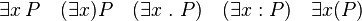$\exists{x}\, P \quad (\exists{x}) P \quad (\exists x \ . \ P) \quad (\exists x : P) \quad \exists{x}(P)$

All of these variations also apply to universal quantification. Other variations for the universal quantifier are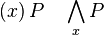$(x) \, P \quad \bigwedge_{x} P$

Early twentieth-century documents do not use the ∀ symbol. The typical notation was (x)P to express "for all x, P," and "(∃x)P" for "there exists x such that P." The ∃ symbol was coined by Giuseppe Peano around 1890. Later, around 1930, Gerhard Gentzen introduced the ∀ symbol to represent universal quantification. Frege's Begriffsschrift used an entirely different notation, which did not include an existential quantifier at all; ∃x P was always represented instead with the Begriffsschrift equivalent of ∀x P.

### Formal semantics

Now we illustrate the way the quantifiers are treated in formal languages by taking the example of the first-order logic. The readers are referred to predicate calculus for more details.

An interpretation for first-order predicate calculus assumes as given a domain of individuals X. A formula A whose free variables are x1, ..., xn is interpreted as a boolean-valued function F(v1, ..., vn) of n arguments, where each argument ranges over the domain X. Boolean-valued means that the function assumes one of the values T (interpreted as truth) or F (interpreted as falsehood). The interpretation of the formula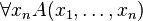$\forall x_n A(x_1, \ldots , x_n)$

is the function G of n-1 arguments such that G(v1, ...,vn-1) = T if and only if F(v1, ..., vn-1, w) = T for every w in X. If F(v1, ..., vn-1, w) = F for at least one value of w, then G(v1, ...,vn-1) = F. Similarly the interpretation of the formula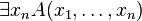$\exists x_n A(x_1, \ldots , x_n)$

is the function H of n-1 arguments such that H(v1, ...,vn-1) = T if and only if F(v1, ...,vn-1, w) = T for at least one w and H(v1, ..., vn-1) = F otherwise.

The semantics for uniqueness quantification requires first-order predicate calculus with equality. This means there is given a distinguished two-placed predicate "="; the semantics is also modified accordingly so that "=" is always interpreted as the two-place equality relation on X. The interpretation of$\exists ! x_n A(x_1, \ldots , x_n)$

then is the function of n-1 arguments, which is the logical and of the interpretations of$\exists x_n A(x_1, \ldots , x_n)$$\forall y,z \left\{ A(x_1, \ldots ,x_{n-1}, y) \wedge A(x_1, \ldots ,x_{n-1}, z) \implies y = z \right\}$

### History of formalization

Term logic treats quantification in a manner that is closer to natural language, and also less suited to formal analysis. Aristotelian logic treated All', Some and No in the first century B.C.E., in an account also touching on the alethic modalities.

The first variable-based treatment of quantification was Gottlob Frege's 1879 Begriffsschrift. To universally quantify a variable, Frege would make a dimple in an otherwise straight line appearing in his diagrammatic formulas, then write the quantified variable over the dimple. Frege did not have a specific notation for existential quantification, instead using the equivalent of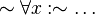$\sim\forall x:\sim\ldots$. Frege's treatment of quantification went largely unremarked until Bertrand Russell's 1903 Principles of Mathematics.

Meanwhile, Charles Sanders Peirce and his student O. H. Mitchell independently invented the existential as well as the universal quantifier, in work culminating in Peirce (1885). Peirce and Mitchell wrote Πx and Σx where we now write ∀x and ∃x. This notation can be found in the writings of Ernst Schroder, Leopold Loewenheim, Thoralf Skolem, and Polish logicians into the 1950s. It is the notation of Kurt Goedel's landmark 1930 paper on the completeness of first-order logic, and 1931 paper on the incompleteness of Peano arithmetic. Peirce's later existential graphs can be seen as featuring tacit variables whose quantification is determined by the shallowest instance. Peirce's approach to quantification influenced Ernst Schroder, William E. Johnson, and all of Europe via Giuseppe Peano. Pierce's logic has attracted fair attention in recent decades by those interested in heterogeneous reasoning and diagrammatic inference.

Peano notated the universal quantifier as (x). Hence "(x)φ" indicated that the formula φ was true for all values of x. He was the first to employ, in 1897, the notation (∃x) for existential quantification. The Principia Mathematica of Whitehead and Russell employed Peano's notation, as did Quine and Alonzo Church throughout their careers. Gentzen introduced the ∀ symbol 1935 by analogy with Peano's ∃ symbol. ∀ did not become canonical until the 1950s.